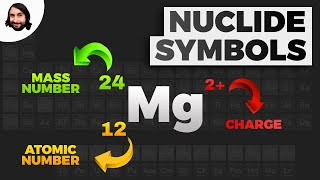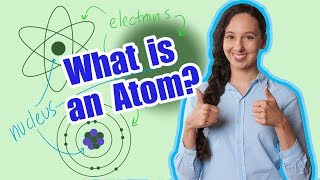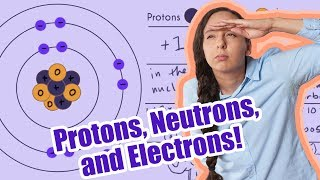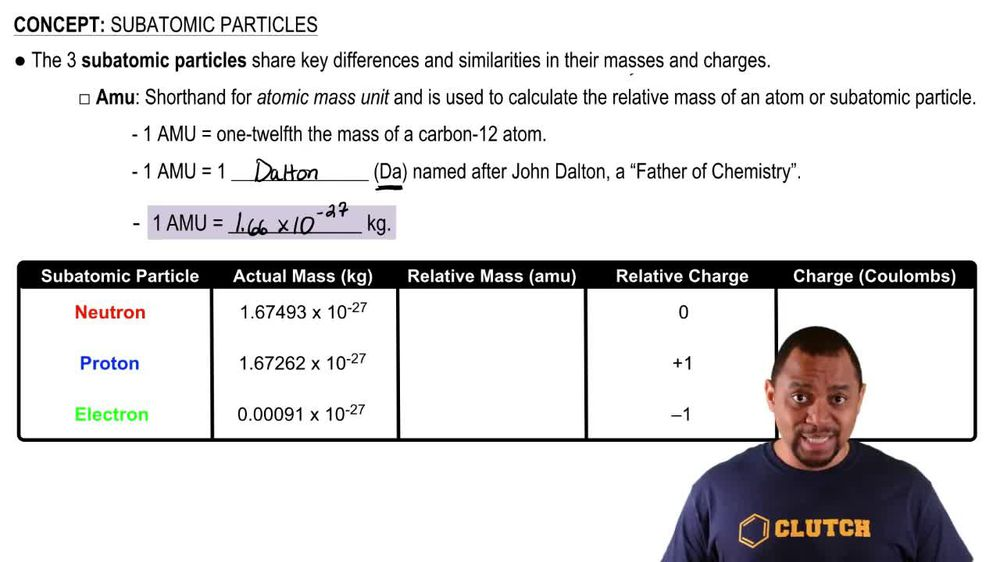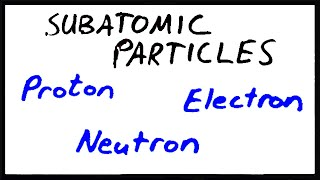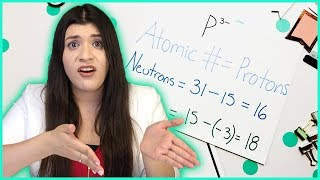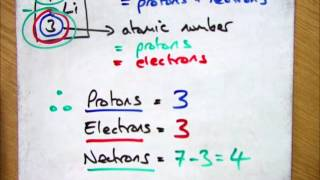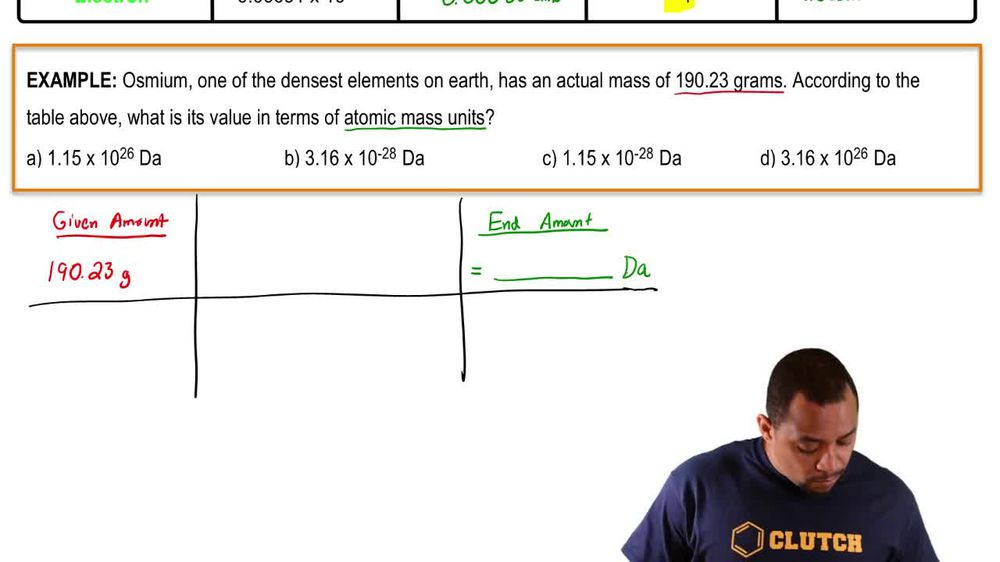Start typing, then use the up and down arrows to select an option from the list.
1. 2. Atoms & Elements2. Subatomic Particles
Problem

# Which statements about subatomic particles are false? a. Protons and electrons have charges of the same magnitude but opposite sign. b. Protons have about the same mass as neutrons. c. Some atoms don’t have any protons. d. Protons and neutrons have charges of the same magnitude but opposite signs.

Relevant Solution4m
Play a video:
hey everyone in this example, we need to identify the incorrect statement about subatomic particles. So statement A begins by saying that protons are attracted to neutrons. Now we wouldn't necessarily use the word attracted. However, in this case, I believe that statement A is referring to the fact that in the nucleus of an atom we have within that nucleus we have our protons and we have our neutrons that are held together within the nucleus by the nuclear or I should say rather the nuclear strong force and the nuclear strong force is what is keeping these protons and neutrons contained within the nucleus. So we would actually go ahead and we consider statement as true because I believe that it's referring to the fact that protons and neutrons are contained within the nucleus of a given atom. So moving onto statement be, neutrons are much heavier than electrons. So we want to recall the trend where neutrons are actually going to have the largest mass. So this is going from mass from largest to smallest. So we would recall that neutrons have the largest mass. We would recall that after neutrons we have a greater mass. We're sorry, a lower mass contributed from protons which have just a smaller mass than neutrons. And lastly after protons, we have a significantly smaller mass coming from our electrons. And so we would agree with the statement that neutrons are much heavier than electrons because electrons have the smallest mass. And so we would consider statement be true. Moving onto statement see it says neutrons have half the mass of a proton. So here we're going to reference the specific weight of a neutron and a proton. So we want to recall that a neutron has a weight that is equal to 1.67493 times 10 to the negative 27 power kilograms. Whereas protons have a weight that is equal to 1. to 62 Times 10 to the -27 Power kg. And as you can see, neutrons differ by just a figure above 2 62. Whereas neutrons have 493 in the ending of the given weight, we still have the same um Power of 10 that are neutrons and protons are being multiplied by but the weight of a neutron is just a bit above to 62. And so we would say that neutrons don't necessarily have half the mass of a proton, but they're only just a bit above the mass of a proton. So we would actually consider statements as false. So this could be a good answer choice that we can agree with. So let's actually highlight that yellow. Since we do want to correct or choose the incorrect statement. Moving on to answer choice D it says a neutral atom has an equal number of protons and electrons. So we do want to recall that protons are going to be positively charged and we want to recall that electrons are going to be negatively charged. And so with that being said, if we have a given atom with the number of protons that are equal to the number of electrons, I would agree that the positive and negative charges from the protons and the electrons would neutralize one another, therefore giving us a neutral atom overall. And so we would agree that statement D is actually a correct statement. So to complete this example, the only correct answer choice that is incorrect about subatomic particles would be statement see which says that neutrons have half the mass of a proton. We know that it's false because we compared the two masses between a neutron and proton over here. So this completes this example as our final answer. If you have any questions, please leave them down below and I will see everyone in the next practice video.